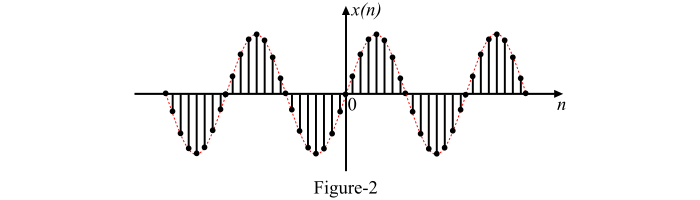# Continuous-Time Vs Discrete-Time Sinusoidal Signal

A sinusoidal function or sinusoidal signal is a function that describes a smooth periodic oscillation.

## Continuous-Time Sinusoidal Signal

A sinusoidal signal which is defined for every instant of time is called continuous-time sinusoidal signal. The continuous time sinusoidal signal is given as follows −

𝑥(𝑡) = 𝐴 sin(𝜔𝑡 + 𝜑) = 𝐴 sin(2𝜋𝑓𝑡 + 𝜑)

Where,

• A is the amplitude of the signal. That is the peak deviation of the signal from zero.
• ω=2πf is the angular frequency in radians per seconds.
• f is the frequency of the signal in Hz.
• φ is the phase angle in radians.

All the continuous-time sinusoidal signals are periodic signal. The time period (T) of a continuous-time sinusoidal signal is given by,

$$\mathrm{T = \frac{2\pi}{\omega }=\frac{1}{f}}$$

The graphical representation or waveform of a continuous time sinusoidal signal x(t) is shown in Figure-1.## Discrete-Time Sinusoidal Signal

A sinusoidal signal which is defined only at discrete instants of time is called discrete-time sinusoidal signal. The discrete-time sinusoidal signal is given as follows −

𝑥(𝑛) = 𝐴 sin(𝜔𝑛 + 𝜑) = 𝐴 sin(2𝜋𝑓𝑛 + 𝜑)

Where,

• A is the amplitude of the signal.

• ω=2πf is the angular frequency in radians per seconds.

• f is the frequency of the signal in Hz.

• φ is the phase angle in radians.

• n is an integer.

The time period of the discrete-time sinusoidal signal is given by,

$$\mathrm{N = \frac{2\pi}{\omega }m}$$

Where, N and m are integers.

The discrete-time sinusoidal sequences may or may not be periodic depending on the values of angular frequency (ω). For a discrete-time sinusoidal signal to be periodic, the angular frequency (ω) must be a rational multiple of 2π.

The graphical representation of a discrete-time sinusoidal signal x(n) is shown in Figure-2.## Why Sinusoidal Signal is so Important?

The sinusoidal signals or sinusoidal functions are very important in both electrical and electronics engineering. According to the Fourier series theory, any periodic signal can be written in terms of sinusoidal signals (i.e., sine and cosine signals) of different frequencies. Thus, a complex signal can be converted into simple sine and cosine signals and hence the analysis of the signals becomes easy.

Also, in the mathematical operations of signals, the sinusoidal signal is the only signal whose summation, multiplication, differentiation or integration be also a sinusoidal signal. For example, in transformers the output voltage is the time derivative of magnetic flux. The magnetic flux is itself a time derivative of input voltage. But we need same voltage signal at both input and output end. The only signals that satisfy this condition are sine and cosine signals (or sinusoidal signals).